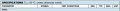# power loss of mosfet

#### MAINAHNOUD

Joined Jul 24, 2019
67
is the power loss of mosfet equal current^2 * Ron?
so, how can i calculate the power loss on it?

#### ronsimpson

Joined Oct 7, 2019
2,712
Power = current^2 * Resistor
current^2=current * current=current squared
This is only the DC loss.

•MAINAHNOUD

#### dl324

Joined Mar 30, 2015
15,536
is the power loss of mosfet equal current^2 * Ron?
$$P = IV = I^2R = \frac{V^2}{R}$$

Since you don't know R, current time voltage would be more reliable.

#### MAINAHNOUD

Joined Jul 24, 2019
67
Power = current^2 * Resistor
current^2=current * current=current squared
This is only the DC loss.
So, what is the other power loss?

#### MAINAHNOUD

Joined Jul 24, 2019
67
$$P = IV = I^2R = \frac{V^2}{R}$$

Since you don't know R, current time voltage would be more reliable.
R is on datasheet right?
Power loss is due to resistance right?

#### dl324

Joined Mar 30, 2015
15,536
R is on datasheet right?
The datasheet doesn't give you a way to determine ON resistance unless you're operating at the conditions they specified.

This is for IRF530:If you're operating at Vgs=10V and Id=8.4A, the Rmax is 0.15 ohms. It could be less, but they don't say how much less. If you operate at different conditions, resistance will be different.

#### dl324

Joined Mar 30, 2015
15,536